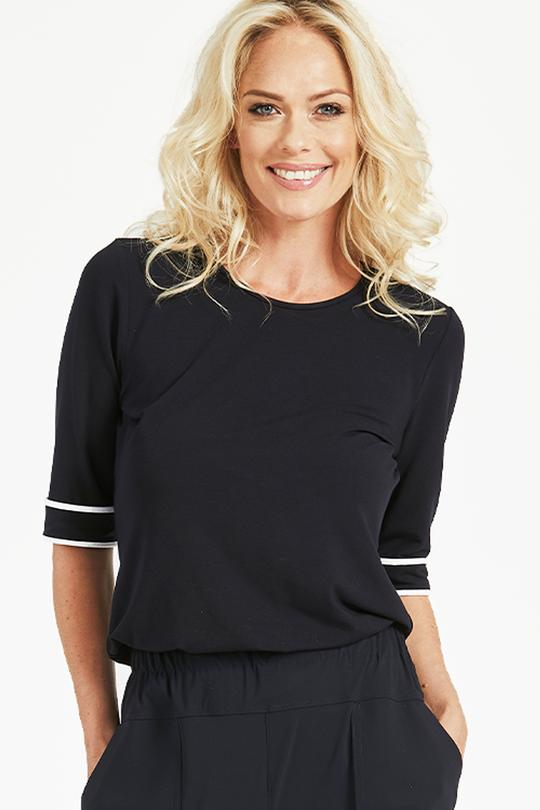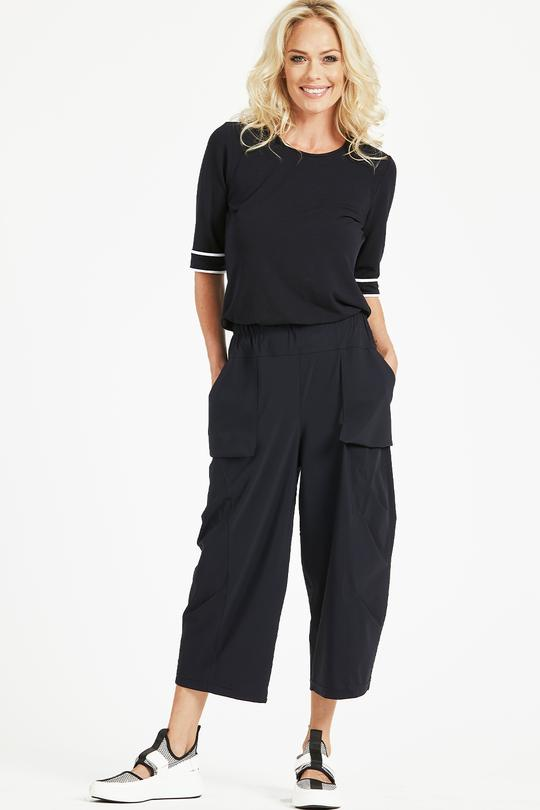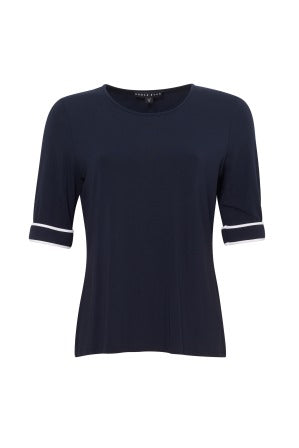Love it? Get it now, pay later withFREE SHIPPING orders \$200+ in NZ
• FREE SHIPPING orders \$200+ in NZ
• MY CART    0# Paula Ryan Slim Fit Half Sleeve Crew Neck In Navy

\$290.00

Size
##### Quantity

Measurements (cm):

• Neck to hem(Back): XS=59.8 S=60.2 M=61 L=61.8 XL=82.6 2XL=63.4 XL=64.2.
• Bust Circumference: XS=91 S=94 M=100 L=106 XL=112 2XL=118 3XL=124.
• Waist:XS=83 S=86 M=92 L=98 XL=104 2XL=110 3XL=116.
• Hem Width: XS=94 S=97 M=103 L=109 XL=115 2XL=121 3XL=127.
• Shoulder to Shoulder: XS=35.1 S= 35.9 M= 37.5 L= 39.1 XL= 40.7 2XL= 42.3 3XL= 43.9
• Sleeve (Head to Hem): XS=27.3 S=27.7 M= 28.5 L=29.3 XL=30.1 2XL=30.9 3XL=31.7.

Fabric: 90% MicroModal 10% Lycra

Style no. 8262